SBOSA75B July   2021  – November 2021

PRODUCTION DATA

1. Features
2. Applications
3. Description
4. Revision History
5. Device Comparison Table
6. Pin Configuration and Functions
7. Specifications
8. Detailed Description
1. 8.1 Overview
2. 8.2 Functional Block Diagram
3. 8.3 Feature Description
4. 8.4 Device Functional Modes
9. Application and Implementation
1. 9.1 Application Information
2. 9.2 Typical Applications
1. 9.2.1 Resistive-Bridge Pressure Sensor
2. 9.2.2 Supporting High Common-Mode Voltage in PLC Input Modules
10. 10Power Supply Recommendations
11. 11Layout
12. 12Device and Documentation Support
1. 12.1 Device Support
1. 12.1.1 Development Support
2. 12.2 Documentation Support
4. 12.4 Support Resources
6. 12.6 Electrostatic Discharge Caution
7. 12.7 Glossary
13. 13Mechanical, Packaging, and Orderable Information

• D|8
• DGK|8

## 7.6 Typical Characteristics

at TA = 25°C, VS = ±15 V, RL = 10 kΩ, CL = 0 pF, VCM = VREF = 0 V, and G = 1 (unless otherwise noted)

Table 7-1 Table of Graphs
FIGURE TITLEFIGURE NUMBER
Typical Distribution Graphs
Typical Distribution of Input Stage Offset VoltageFigure 7-1
Typical Distribution of Input Stage Offset Voltage DriftFigure 7-2
Typical Distribution of Output Stage Offset VoltageFigure 7-3
Typical Distribution of Output Stage Offset Voltage DriftFigure 7-4
Typical Distribution of Inverting Input Bias CurrentFigure 7-5
Typical Distribution of Noninverting Input Bias CurrentFigure 7-6
Typical Distribution of Input Offset CurrentFigure 7-7
Typical CMRR Distribution, G = 1Figure 7-8
Typical CMRR Distribution, G = 10Figure 7-9
Typical Gain Error DistributionFigure 7-10
vs Temperature Graphs
Input Stage Offset Voltage vs TemperatureFigure 7-11
Output Stage Offset Voltage vs TemperatureFigure 7-12
Input Bias Current vs TemperatureFigure 7-13
Input Offset Current vs TemperatureFigure 7-14
CMRR vs Temperature, G = 1Figure 7-15
CMRR vs Temperature, G = 10Figure 7-16
Gain Error vs Temperature, G = 1Figure 7-17
Gain Error vs Temperature, G = 100Figure 7-18
Supply Current vs TemperatureFigure 7-19
AC Performance Graphs
Closed-Loop Gain vs FrequencyFigure 7-20
CMRR vs Frequency (RTI)Figure 7-21
CMRR vs Frequency (RTI, 1-kΩ source imbalance)Figure 7-22
Positive PSRR vs Frequency (RTI)Figure 7-23
Negative PSRR vs Frequency (RTI)Figure 7-24
Voltage Noise Spectral Density vs Frequency (RTI)Figure 7-25
Current Noise Spectral Density vs Frequency (RTI)Figure 7-26
0.1-Hz to 10-Hz RTI Voltage NoiseFigure 7-27
0.1-Hz to 10-Hz RTI Voltage Noise, G = 1000Figure 7-28
Small-Signal Response, G = 1Figure 7-29
Small-Signal Response, G = 10Figure 7-30
Small-Signal Response, G = 100Figure 7-31
Small-Signal Response, G = 1000Figure 7-32
Large-Signal Step ResponseFigure 7-34
Settling Time vs Step SizeFigure 7-35
Large-Signal Frequency ResponseFigure 7-36
Closed-Loop Output Impedance vs FrequencyFigure 7-37
Input and Output Voltage Graphs
Input Current vs Input OvervoltageFigure 7-38
Gain Nonlinearity, G = 1Figure 7-39
Gain Nonlinearity, G = 10Figure 7-40
Gain Nonlinearity, G = 100Figure 7-41
Gain Nonlinearity, G = 1000Figure 7-42
Positive Input Bias Current vs Common‑Mode Voltage (VS–)Figure 7-43
Positive Input Bias Current vs Common‑Mode Voltage (VS+)Figure 7-44
Negative Input Bias Current vs Common‑Mode Voltage (VS–)Figure 7-45
Negative Input Bias Current vs Common‑Mode Voltage (VS+)Figure 7-46
Offset Voltage vs Common-Mode Voltage, VS = 30 VFigure 7-47
Offset Voltage vs Common-Mode Voltage, VS = 2.7 VFigure 7-48
Positive Output Voltage Swing vs Output Current, VS = 30 VFigure 7-49
Negative Output Voltage Swing vs Output Current, VS = 30 VFigure 7-50
Positive Output Voltage Swing vs Output Current, VS = 2.7 VFigure 7-51
Negative Output Voltage Swing vs Output Current, VS = 2.7 VFigure 7-52
Input Common-Mode Voltage vs Output Voltage, VS = 2.7 V, G = 1Figure 7-53
Input Common-Mode Voltage vs Output Voltage, VS = 2.7 V, G = 1Figure 7-54
Input Common-Mode Voltage vs Output Voltage, VS = 5 V, G = 1Figure 7-55
Input Common-Mode Voltage vs Output Voltage, VS = 5 V, G = 100Figure 7-56
Input Common-Mode Voltage vs Output Voltage, VS = 24 V and VS = 30 V, G = 1Figure 7-57
Input Common-Mode Voltage vs Output Voltage, VS = 24 V and VS = 30 V, G = 10Figure 7-58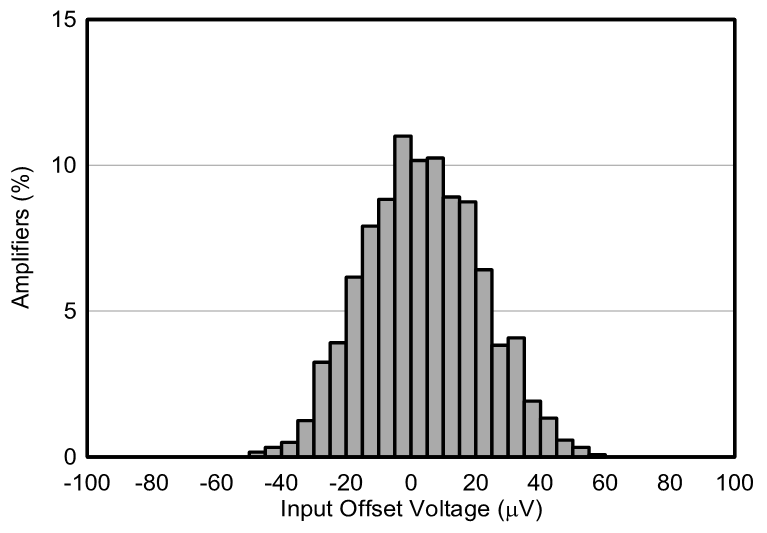N = 1225 Mean = 3.63 μV Std Dev = 18.0 μV
Figure 7-1 Typical Distribution of Input Stage Offset Voltage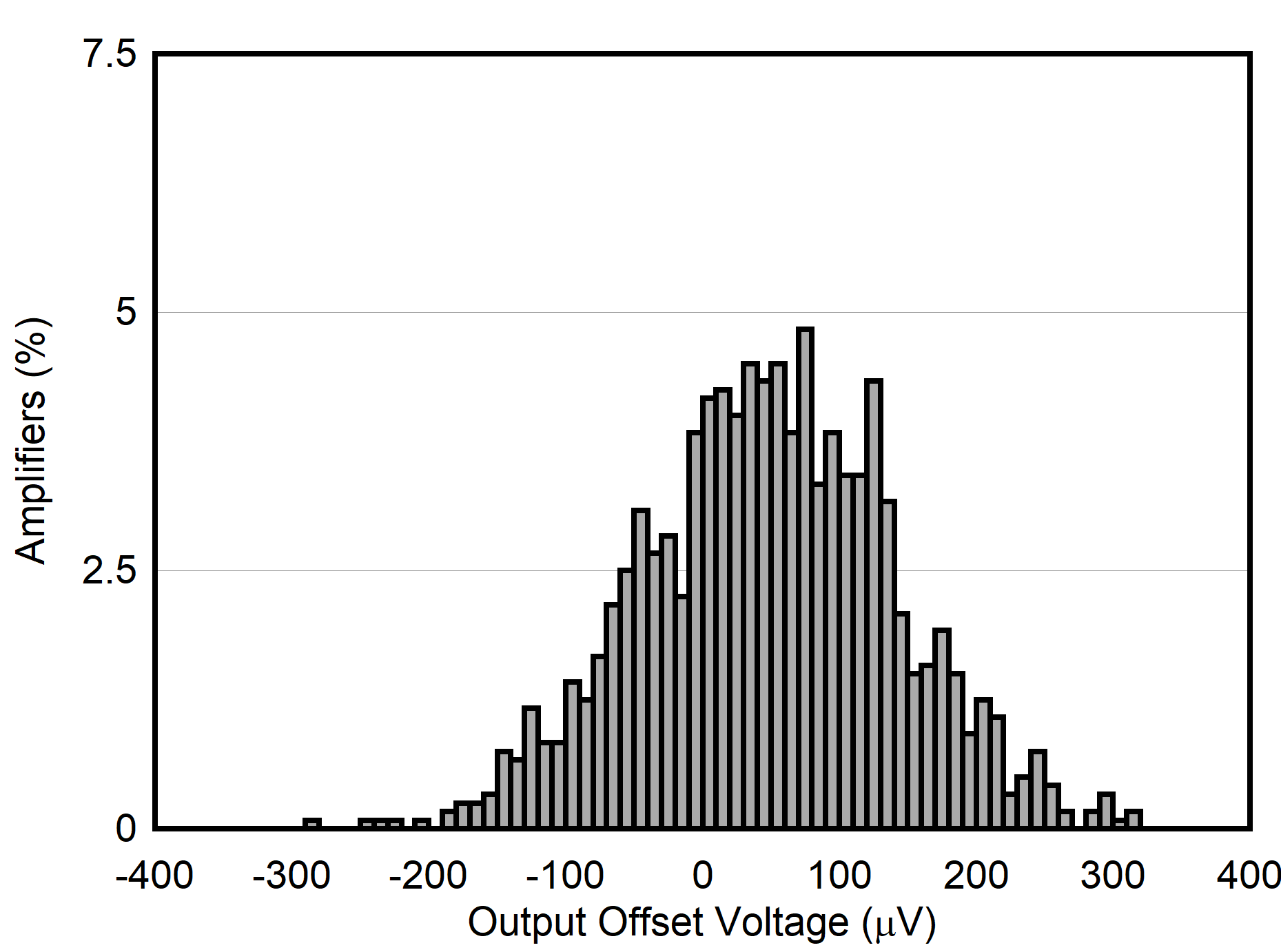N = 1225 Mean = 48.0 μV Std Dev = 92.4 μV
Figure 7-3 Typical Distribution of Output Stage Offset Voltage
 N = 1200 Mean = 1.21 nA Std Dev = 0.384 nA
Figure 7-5 Typical Distribution of Inverting Input Bias Current
 N = 1170 Mean = –0.092 nA Std Dev = 0.35 nA
Figure 7-7 Typical Distribution of Input Offset Current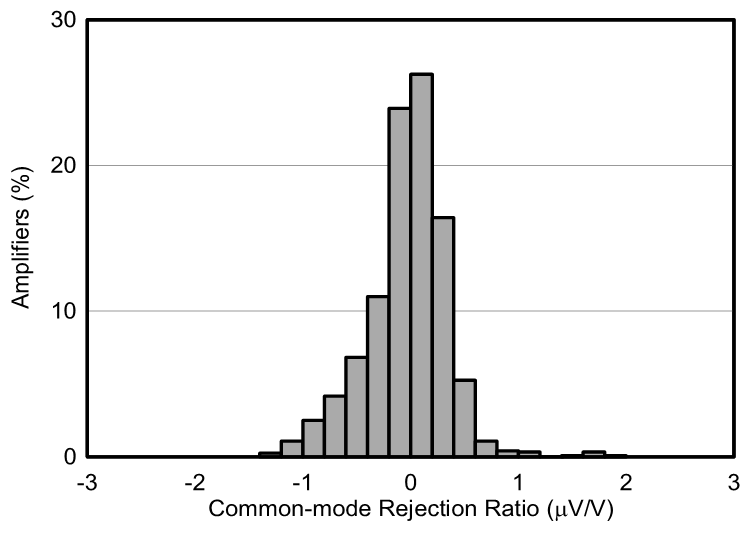N = 1225 Mean = –0.0599 μV/V Std. Dev = 0.710 μV/V G = 10
Figure 7-9 Typical CMRR Distribution
Figure 7-11 Input Stage Offset Voltage vs Temperature
Figure 7-13 Input Bias Current vs Temperature
 G = 1
Figure 7-15 CMRR vs Temperature
 G = 1 Average of 120 units Normalized at +25°C
Figure 7-17 Gain Error vs Temperature
Figure 7-19 Supply Current vs Temperature
Figure 7-21 CMRR vs Frequency (RTI)
Figure 7-23 Positive PSRR vs Frequency (RTI)
Figure 7-25 Voltage Noise Spectral Density vs Frequency (RTI)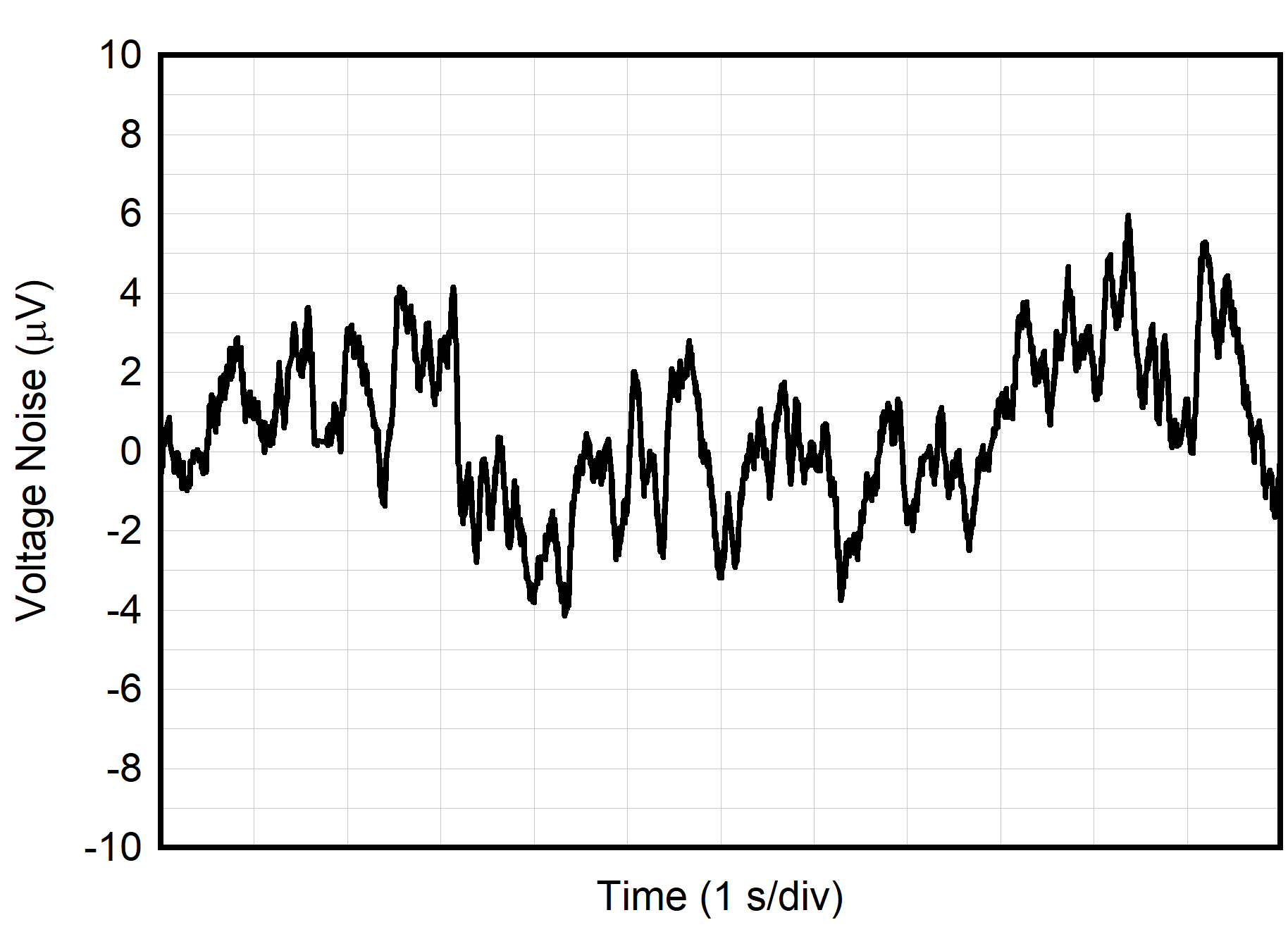Figure 7-27 0.1-Hz to 10-Hz RTI Voltage Noise
 G = 1 RL = 10 kΩ CL = 100 pF
Figure 7-29 Small-Signal Response
 G = 100 RL = 10 kΩ CL = 100 pF
Figure 7-31 Small-Signal Response
Figure 7-33 Overshoot vs Capacitive Loads
Figure 7-35 Settling Time vs Step Size
Figure 7-37 Closed-Loop Output Impedance vs Frequency
Figure 7-39 Gain Nonlinearity
Figure 7-41 Gain Nonlinearity
Figure 7-43 Positive Input Bias Current vs Common‑Mode Voltage (VS–)
Figure 7-45 Negative Input Bias Current vs Common‑Mode Voltage (VS–)
 VS = ±15 V
Figure 7-47 Offset Voltage vs Common-Mode Voltage
 VS = ±15 V
Figure 7-49 Positive Output Voltage Swing vs Output Current
 VS = ±1.35 V
Figure 7-51 Positive Output Voltage Swing vs Output Current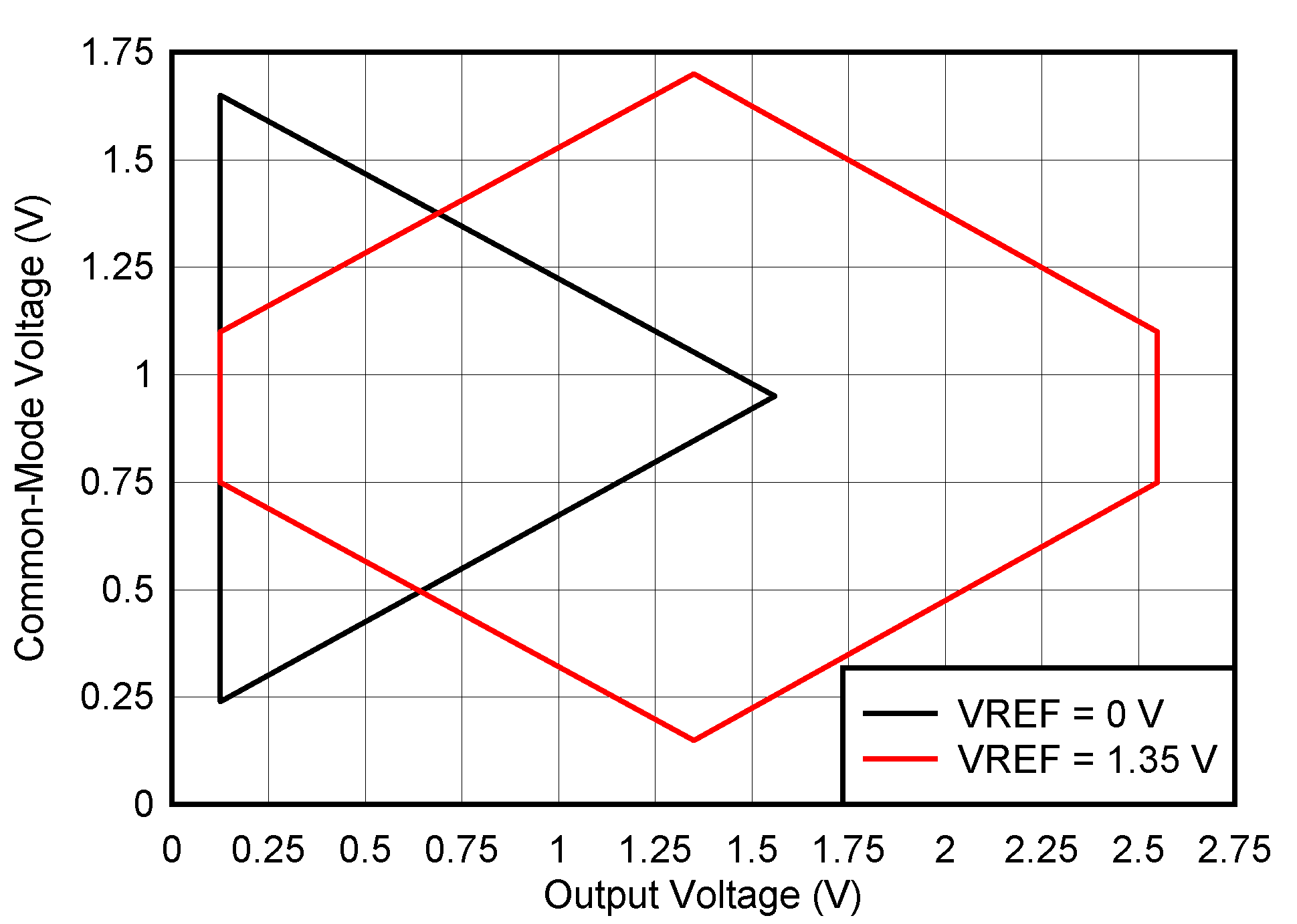VS = 2.7 V G = 1
Figure 7-53 Input Common-Mode Voltage vs Output Voltage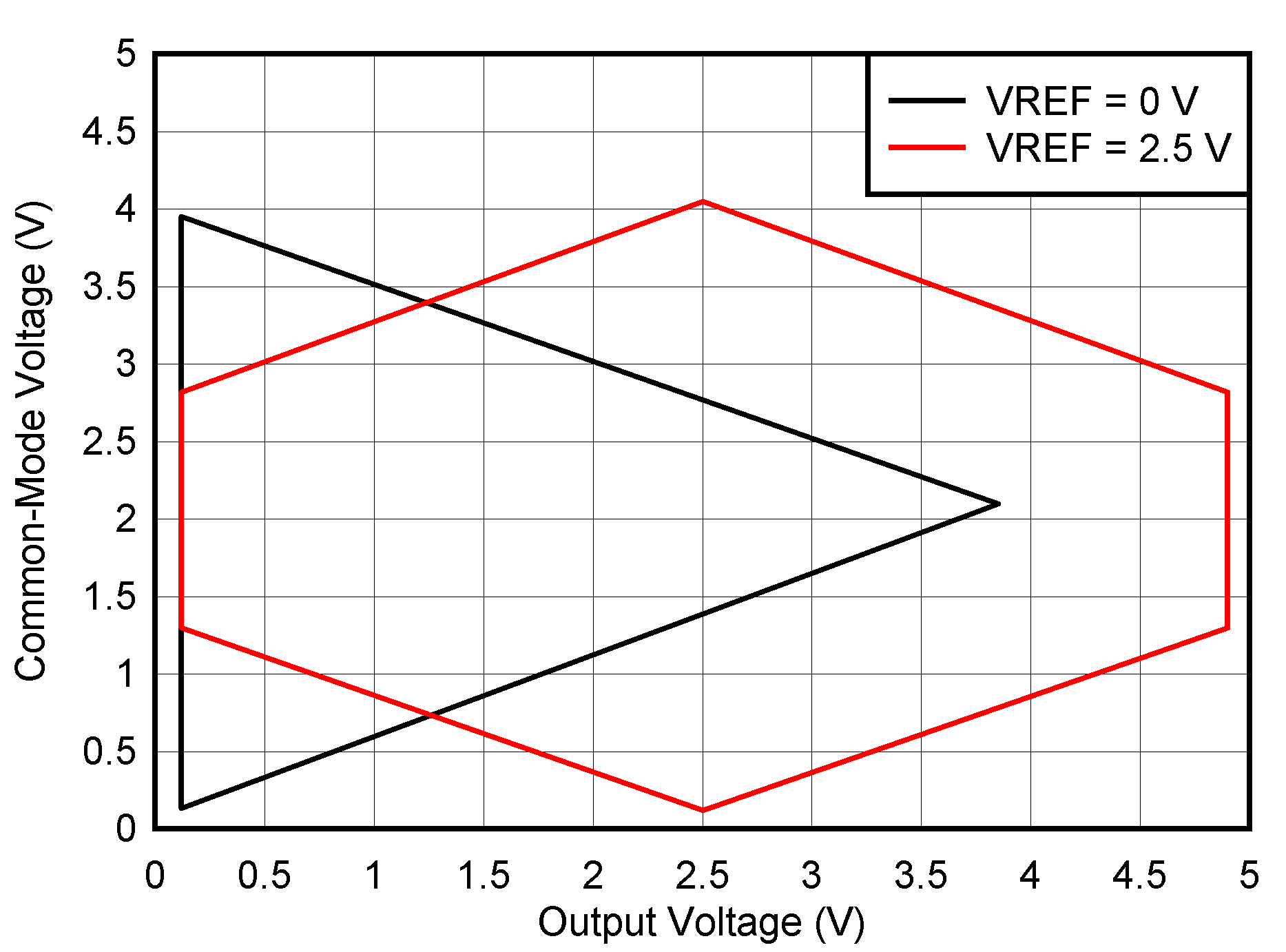VS = 5 V G = 1
Figure 7-55 Input Common-Mode Voltage vs Output Voltage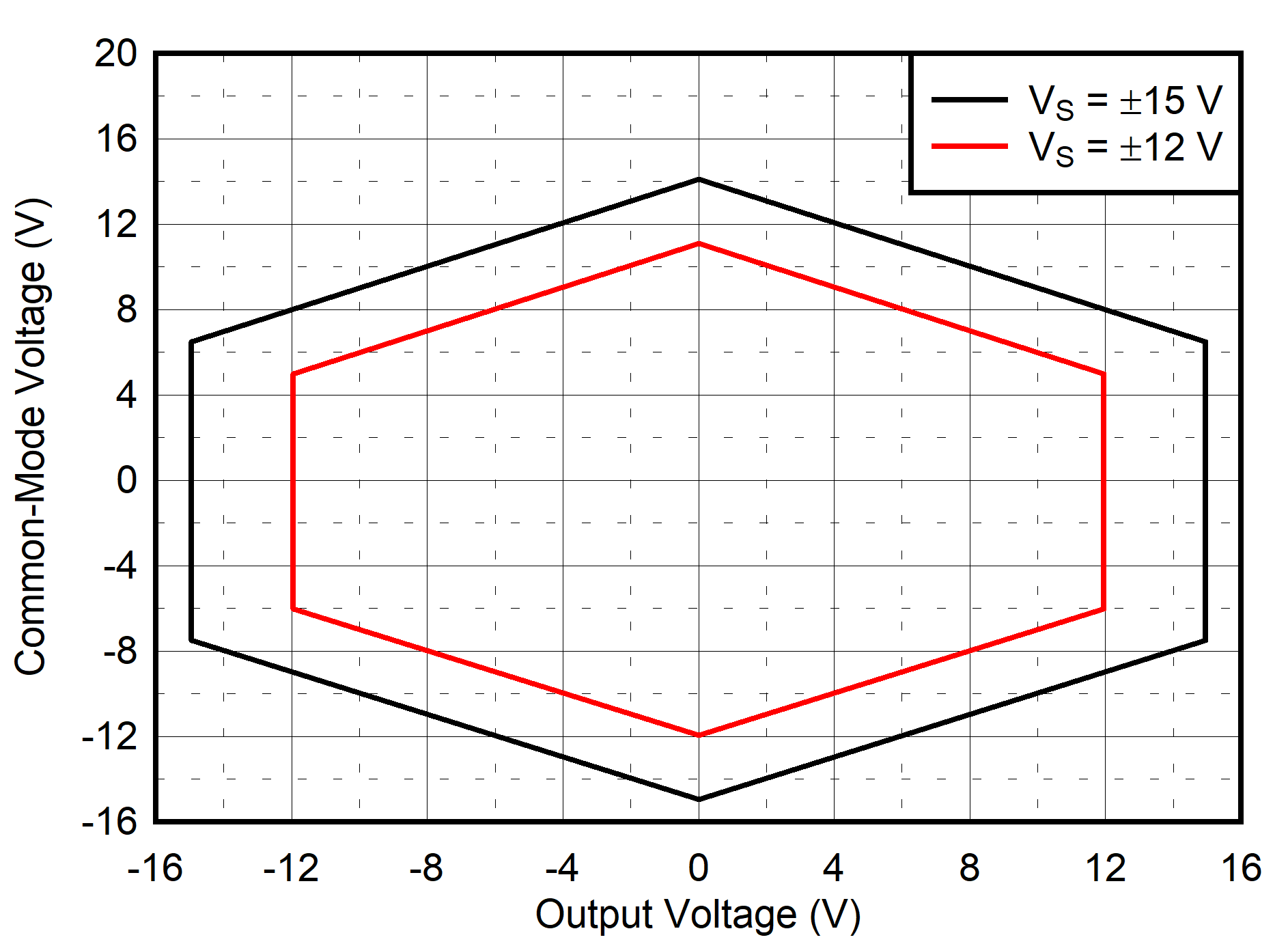G = 1
Figure 7-57 Input Common-Mode Voltage vs Output VoltageN = 30 Mean = –0.024 μV/ºC Std Dev = 0.177 μV//ºC
Figure 7-2 Typical Distribution of Input Stage Offset Voltage Drift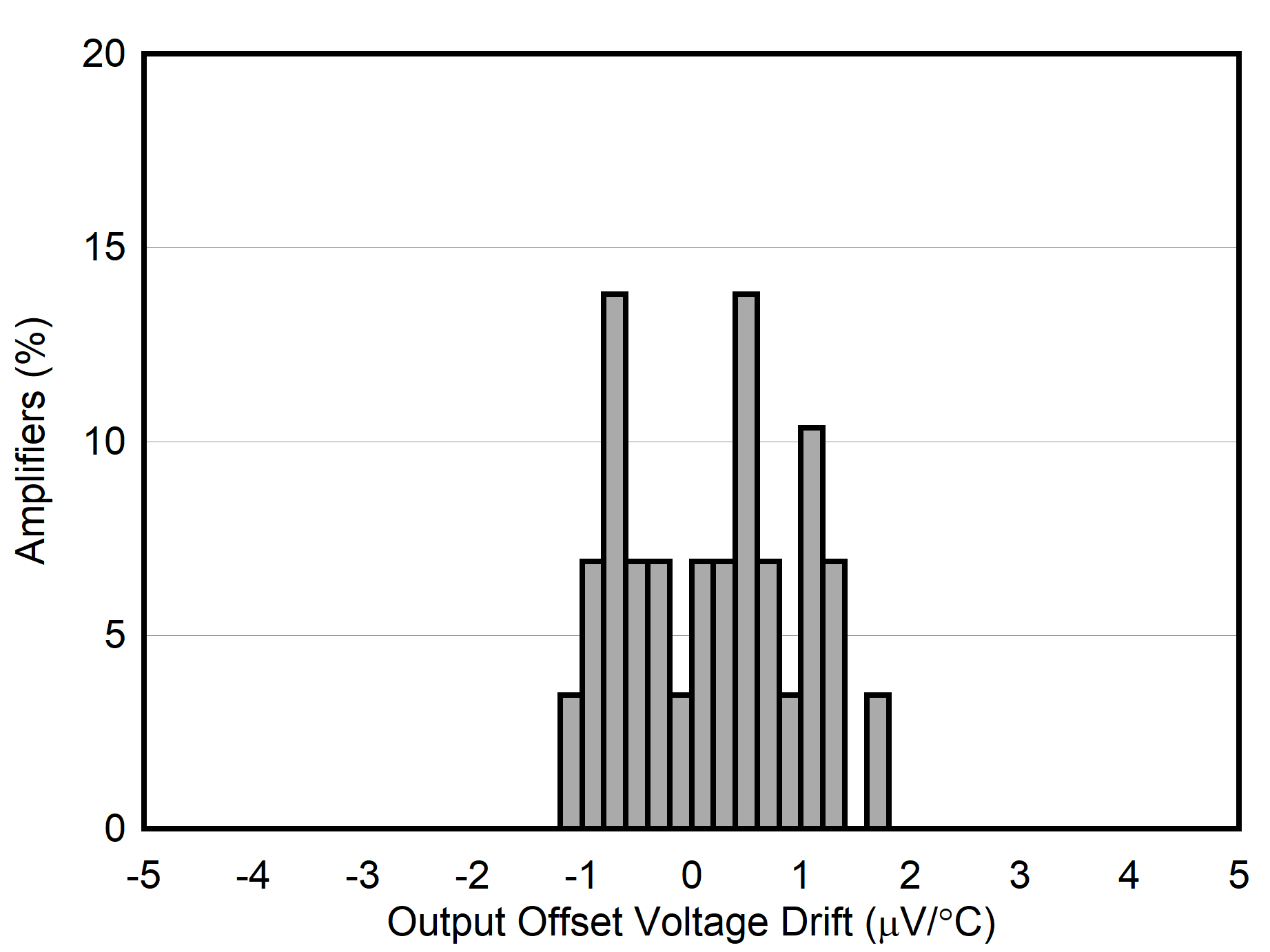N = 30 Mean = 0.17 μV/ºC Std Dev = 0.795 μV/ºC
Figure 7-4 Typical Distribution of Output Stage Offset Voltage Drift
 N = 1200 Mean = 1.11 nA Std Dev = 0.368 nA
Figure 7-6 Typical Distribution of Noninverting Input Bias Current
 N = 1225 Mean = –0.22 μV/V Std Dev = 6.95 μV/V
Figure 7-8 Typical CMRR Distribution
 N = 550 Mean = –0.0334 % Std. Dev = 0.0433 % G = 10
Figure 7-10 Typical Gain Error Distribution
Figure 7-12 Output Stage Offset Voltage vs Temperature
Figure 7-14 Input Offset Current vs Temperature
 G = 10
Figure 7-16 CMRR vs Temperature
 G = 100 Average of 120 units Normalized at +25°C
Figure 7-18 Gain Error vs Temperature
Figure 7-20 Closed-Loop Gain vs Frequency
Figure 7-22 CMRR vs Frequency (RTI, 1-kΩ source imbalance)
Figure 7-24 Negative PSRR vs Frequency (RTI)
Figure 7-26 Current Noise Spectral Density vs Frequency (RTI)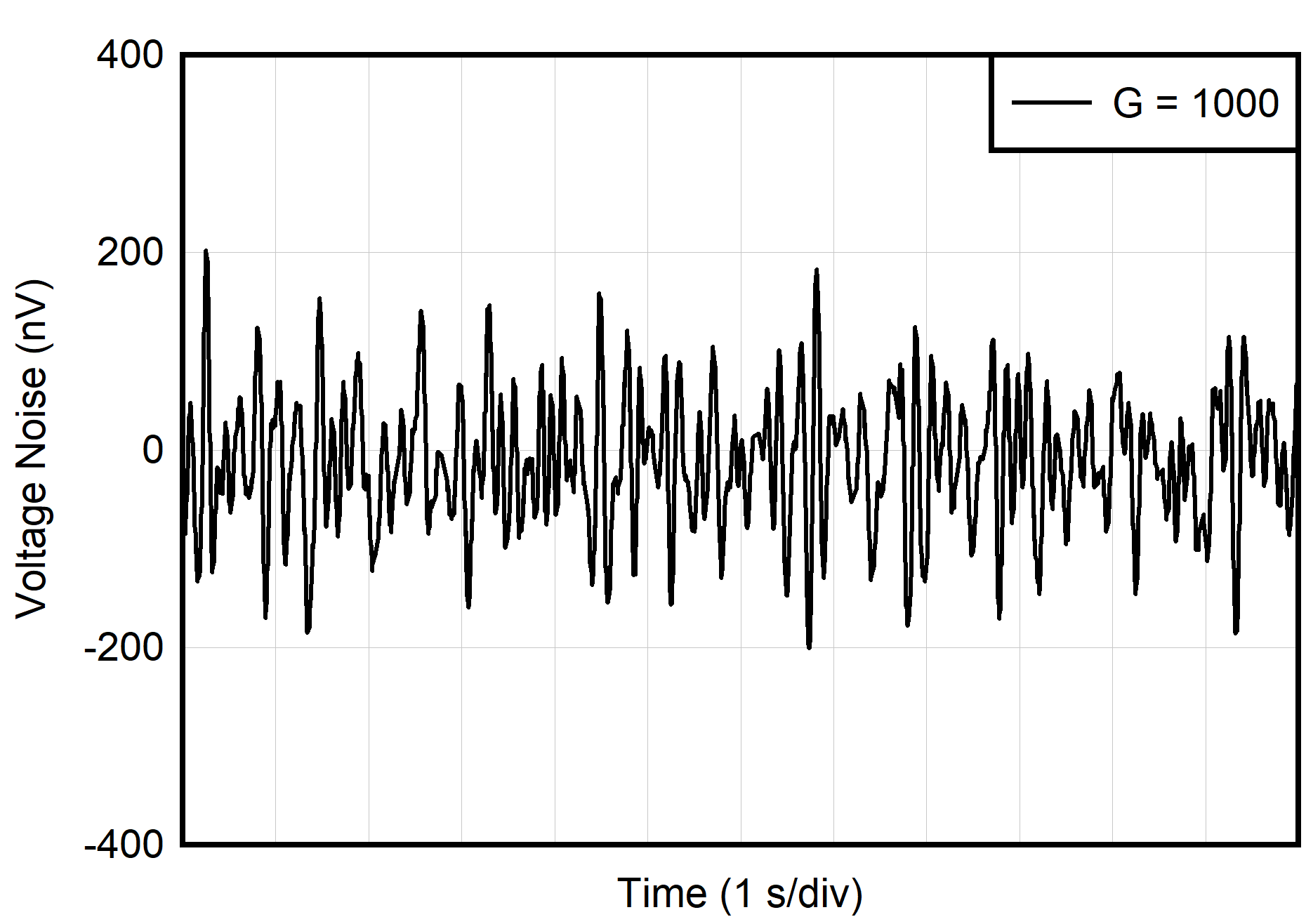Figure 7-28 0.1-Hz to 10-Hz RTI Voltage Noise
 G = 10 RL = 10 kΩ CL = 100 pF
Figure 7-30 Small-Signal Response
 G = 1000 RL = 10 kΩ CL = 100 pF
Figure 7-32 Small-Signal Response
Figure 7-34 Large-Signal Step Response
Figure 7-36 Large-Signal Frequency Response
 VS = ±15 V
Figure 7-38 Input Current vs Input Overvoltage
Figure 7-40 Gain Nonlinearity
Figure 7-42 Gain Nonlinearity
Figure 7-44 Positive Input Bias Current vs Common‑Mode Voltage (VS+)
Figure 7-46 Negative Input Bias Current vs Common‑Mode Voltage (VS+)
 VS = ±1.35 V
Figure 7-48 Offset Voltage vs Common-Mode Voltage
 VS = ±15 V
Figure 7-50 Negative Output Voltage Swing vs Output Current
 VS = ±1.35 V
Figure 7-52 Negative Output Voltage Swing vs Output Current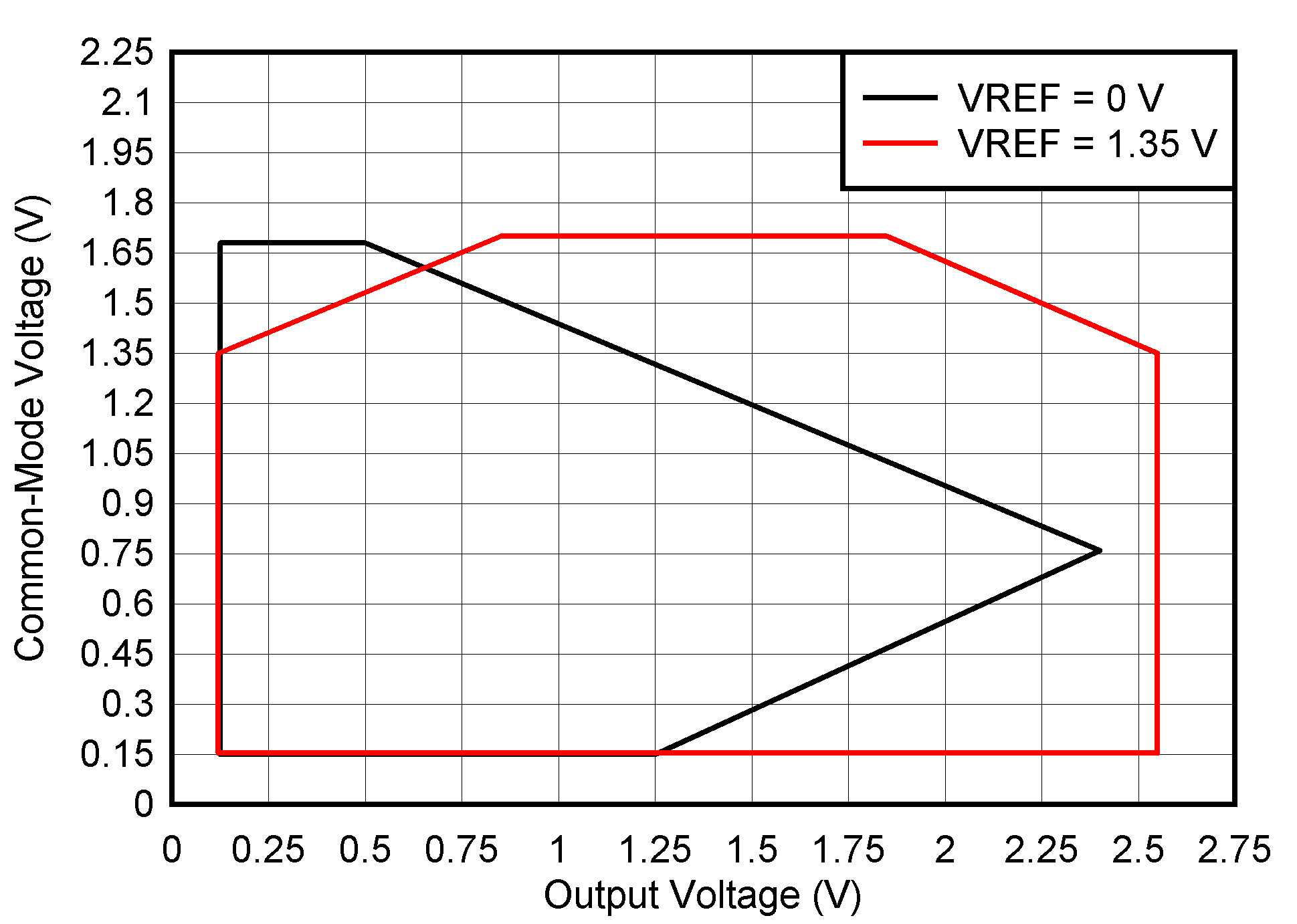VS = 2.7 V G = 100
Figure 7-54 Input Common-Mode Voltage vs Output Voltage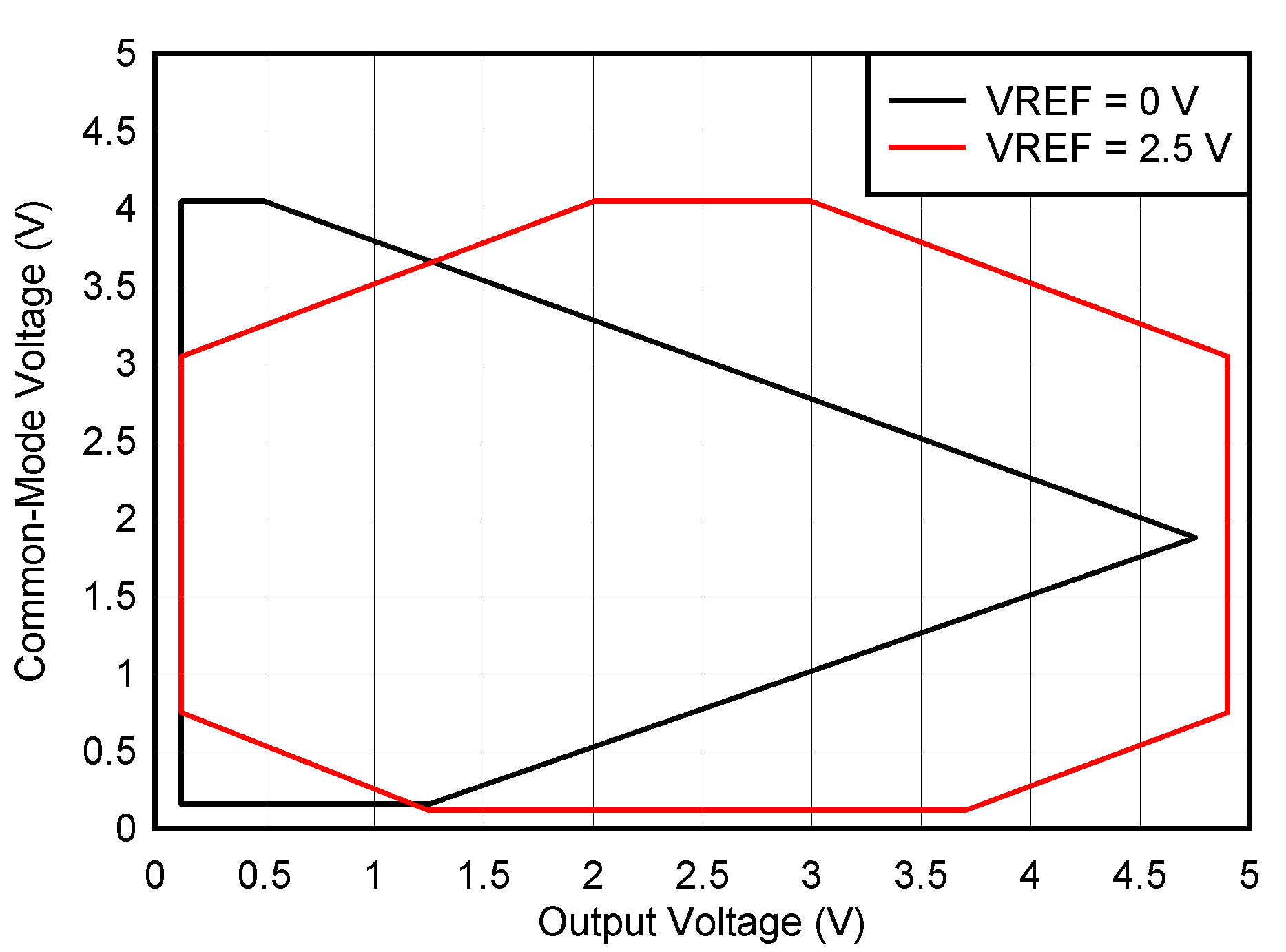VS = 5 V G = 100
Figure 7-56 Input Common-Mode Voltage vs Output Voltage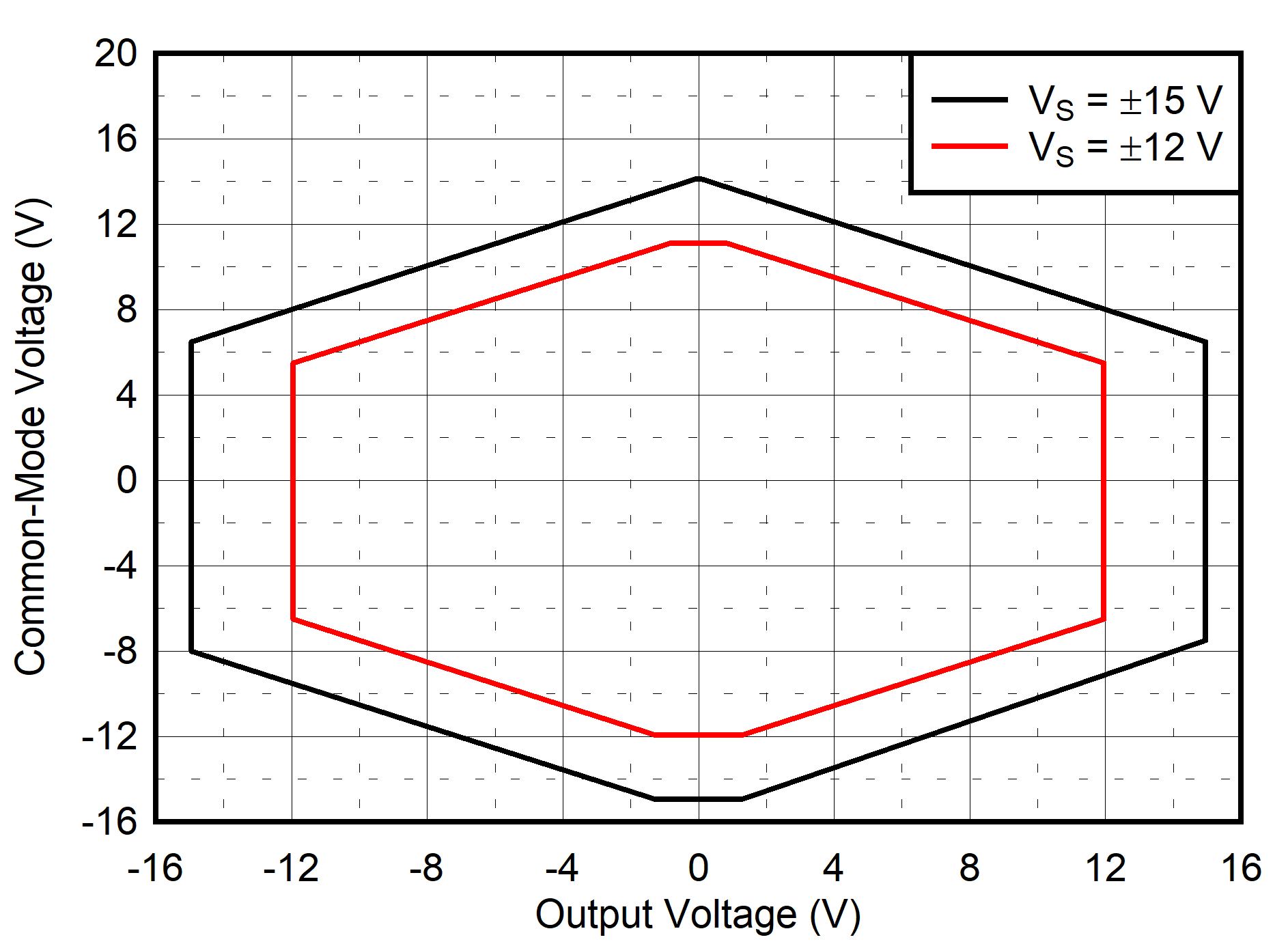G > 10
Figure 7-58 Input Common-Mode Voltage vs Output Voltage